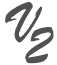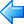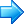Timing functionsindexMpower searchThe following timing functions allow to compute the number of seconds elapsed since a given moment. They are generally used to compute the response time of a program.

1. TimerStart
Use subprocedure timerStart to start computing the elapsed time.
Example:
 ``` * Set timer for calculating execution time C callp TimerStart() ```

1. TimerElapsed
Use subprocedure timerElapsed to receive the number of seconds elapsed since the last timerStart.
Example:
 ``` * Program timing variable D sec s 15p 6 C eval sec = TimerElapsed() C callp updhtmlvar('runtime':%editc(sec:'N')) C callp wrtsection('runtime')```